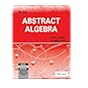Normal view

## Abstract algebra / by David S. Dummit and Richard M. Foorte

Material type:TextPublication details: New Delhi : John wiley & sons. 2012, 2004cEdition: 3rd edDescription: xii, 932 p. : ill. ; 25 cmISBN: 9788126532285Subject(s): Algebra, AbstractDDC classification: 512.02
Contents:
pt. 1. Group theory. Introduction to groups -- Subgroups -- Quotient groups and homomorphisms -- Group actions -- Direct and semidirect products and abelian groups -- Further topics in group theory -- pt. 2. Ring theory. Introduction to rings -- Euclidean domains, principal ideal domains and unique factorization domains -- Polynomial rings -- pt. 3. Modules and vector spaces. Introduction to module theory -- Vector spaces -- Modules over principal ideal domains -- pt. 4. Field theory and Galois theory. Field theory -- Galois theory -- pt. 5. An introduction to commutative rings, algebraic geometry, and homological algebra. Commutative rings and algebraic geometry -- Artinian rings, discrete valuation rings, and Dedekind domains -- Introduction to homological algebra and group cohomology -- pt. 6. Introduction to the representation theory of finite groups. Representation theory and character theory -- Examples and applications of character theory.
Summary: Widely acclaimed algebra text. This book is designed to give the reader insight into the power and beauty that accrues from a rich interplay between different areas of mathematics.
Tags from this library: No tags from this library for this title.Average rating: 0.0 (0 votes)
Item type Current library Call number Status Date due Barcode Item holdsBooks Namal Library
Mathematics
512.02 DUM-A 2012 353 (Browse shelf (Opens below)) Available 353
Total holds: 0
##### Browsing Namal Library shelves, Shelving location: Mathematics Close shelf browser (Hides shelf browser)512 TAB-T 2019 11530 The history of mathematics algebra : Numbers Computers, Philosophers, and the search of meaning / 512 TAB-T 2019 11531 The history of mathematics algebra : Probability and statistics , The science of uncertainty / 512 TAB-T 2019 11532 The history of mathematics algebra : sets, symbols, and the language of thoughts / 512.02 DUM-A 2012 353 Abstract algebra 512.02 FRA-F 2013 377 A first course in abstract algebra 512.02 FRA-F 2013 651 A first course in abstract algebra 512.02 FRA-F 2018 10695 A first course in abstract algebra

Includes index.

pt. 1. Group theory. Introduction to groups -- Subgroups -- Quotient groups and homomorphisms -- Group actions -- Direct and semidirect products and abelian groups -- Further topics in group theory -- pt. 2. Ring theory. Introduction to rings -- Euclidean domains, principal ideal domains and unique factorization domains -- Polynomial rings -- pt. 3. Modules and vector spaces. Introduction to module theory -- Vector spaces -- Modules over principal ideal domains -- pt. 4. Field theory and Galois theory. Field theory -- Galois theory -- pt. 5. An introduction to commutative rings, algebraic geometry, and homological algebra. Commutative rings and algebraic geometry -- Artinian rings, discrete valuation rings, and Dedekind domains -- Introduction to homological algebra and group cohomology -- pt. 6. Introduction to the representation theory of finite groups. Representation theory and character theory -- Examples and applications of character theory.

Widely acclaimed algebra text. This book is designed to give the reader insight into the power and beauty that accrues from a rich interplay between different areas of mathematics.

There are no comments on this title.

to post a comment.
Share